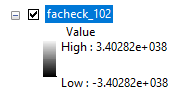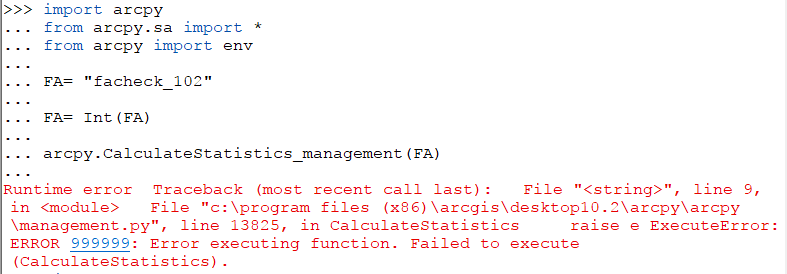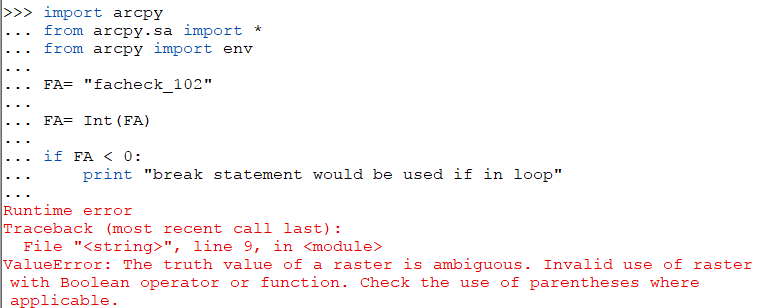# Error with Calculate Statistics

658
2
08-10-2017 12:29 PMNew Contributor III

A program that I created has a loop that runs and it constantly lowers the values of a flow accumulation Raster. There gets to a point where the Raster should hit the value zero or no data. The raster comes out with a positive big number and a negative big number, which messes up the calculate statistics function in the loop. Here it is below.This is an example of the error I would receiveIs there a way I can create an if statement in the loop to check for a raster that looks like this and break away from the loop? Something like this but a statement that would work.1 Solution

Accepted SolutionsbyEsri Esteemed Contributor

You can also use Get Raster Properties—Help | ArcGIS Desktop with the "ALLNODATA" option to test for it.

Example:

``````fa = r'C:\GeoNet\TestNull\myras.tif'
test = arcpy.GetRasterProperties_management(fa, "ALLNODATA")
test = int(test.getOutput(0))
if test == 1:
print "Raster contains all NoData pixels..."
else:
print "Not all pixels are NoData..."‍‍‍‍‍‍‍``````
2 RepliesbyEsri Esteemed Contributor

I assume that you are running this in the Python Window inside a session of ArcMap and the raster is present in the TOC. Otherwise, I guess you wouldn't find a raster only by its name.

You could use a try except to catch any errors:

``````import arcpy
fa = "facheck_102"

try:
fa = arcpy.sa.Int(fa)
arcpy.CalculateStatistics_management(fa)
except Exception as e:
print "ERR:", e‍‍‍‍‍‍‍‍``````byEsri Esteemed Contributor

You can also use Get Raster Properties—Help | ArcGIS Desktop with the "ALLNODATA" option to test for it.

Example:

``````fa = r'C:\GeoNet\TestNull\myras.tif'
test = arcpy.GetRasterProperties_management(fa, "ALLNODATA")
test = int(test.getOutput(0))
if test == 1:
print "Raster contains all NoData pixels..."
else:
print "Not all pixels are NoData..."‍‍‍‍‍‍‍``````